# AQA A Level Chemistry复习笔记1.1.4 Shells and Orbitals

### Electron Shells

#### Shells

• The arrangement of electrons in an atom is called the electron configuration
• Electrons are arranged around the nucleus in principal energy levels or principal quantum shells
• Principal quantum numbers (n) are used to number the energy levels or quantum shells
• The lower the principal quantum number, the closer the shell is to the nucleus
• So, the first shell which is the closest to the nucleus is n = 1

• The higher the principal quantum number, the greater the energy of the shell and the further away from the nucleus

• Each principal quantum number has a fixed number of electrons it can hold
• n = 1 : up to 2 electrons
• n = 2 : up to 8 electrons
• n = 3 : up to 18 electrons
• n = 4 : up to 32 electrons

#### Subshells

• The principal quantum shells are split into subshells which are given the letters s, p and d
• Elements with more than 57 electrons also have an f shell
• The energy of the electrons in the subshells increases in the order s < p < d

• The order of subshells appear to overlap for the higher principal quantum shells as seen in the diagram below: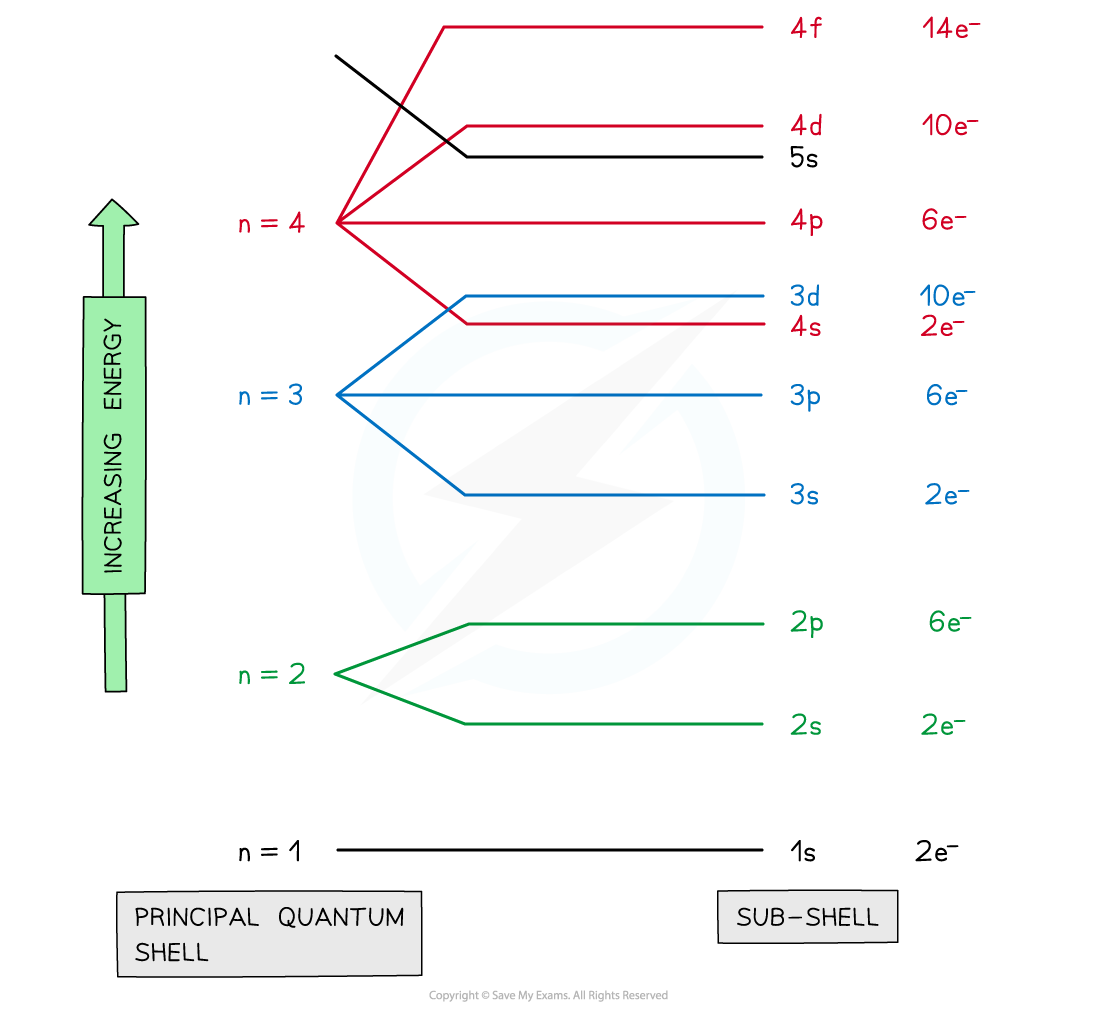Electrons are arranged in principal quantum shells, which are numbered by principal quantum numbers

#### Orbitals

• Subshells contain one or more atomic orbitals
• Orbitals exist at specific energy levels and electrons can only be found at these specific levels, not in between them
• Each atomic orbital can be occupied by a maximum of two electrons

• This means that the number of orbitals in each subshell is as follows:
• s : one orbital (1 x 2 = total of 2 electrons)
• p : three orbitals ( 3 x 2 = total of 6 electrons)
• d : five orbitals (5 x 2 = total of 10 electrons)
• f : seven orbitals (7 x 2 = total of 14 electrons)

• The orbitals have specific 3-D shapes

#### s orbital shape

• The s orbitals are spherical in shape
• The size of the s orbitals increases with increasing shell number
• E.g. the s orbital of the third quantum shell (n = 3) is bigger than the s orbital of the first quantum shell (n = 1)

#### p orbital shape

• The p orbitals have a dumbbell shape
• Every shell has three p orbitals except for the first one (n = 1)
• The p orbitals occupy the x, y and z axes and point at right angles to each other, so are oriented perpendicular to one another
• The lobes of the p orbitals become larger and longer with increasing shell number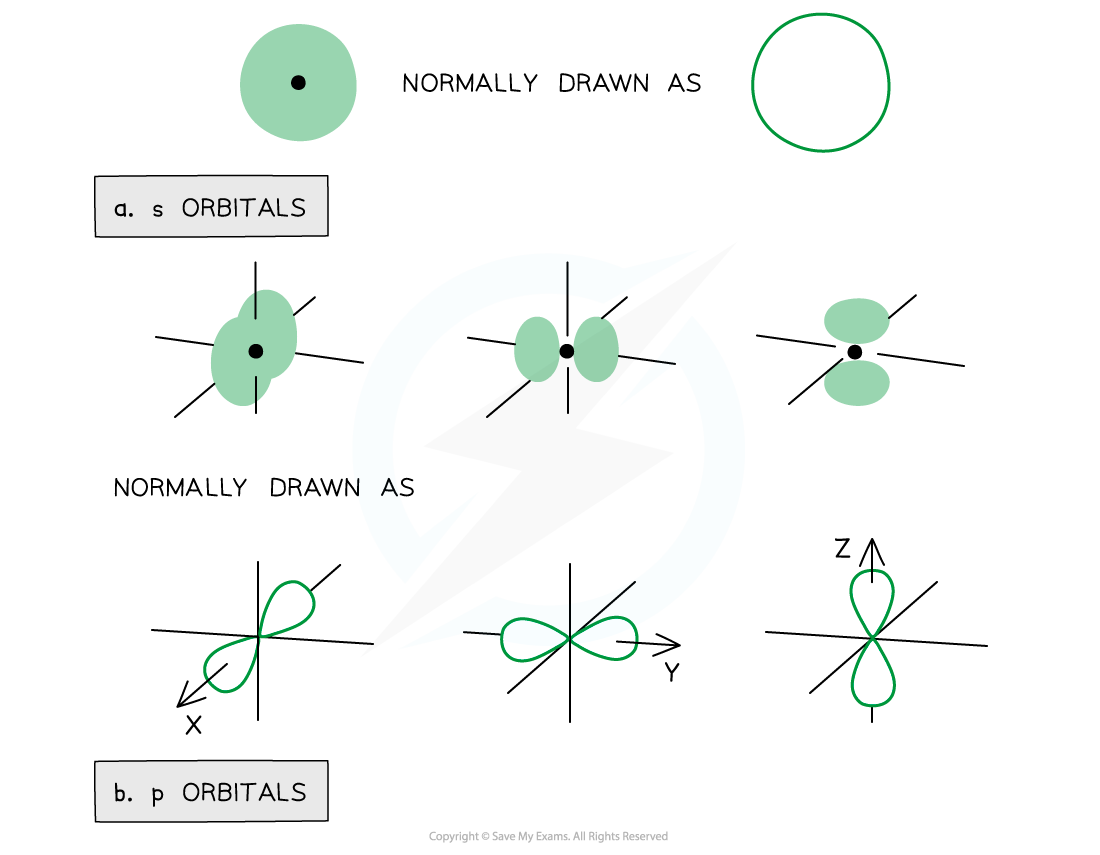Representation of orbitals (the dot represents the nucleus of the atom) showing spherical s orbitals (a), p orbitals containing ‘lobes’ along the x, y and z axis

• Note that the shape of the d orbitals is not required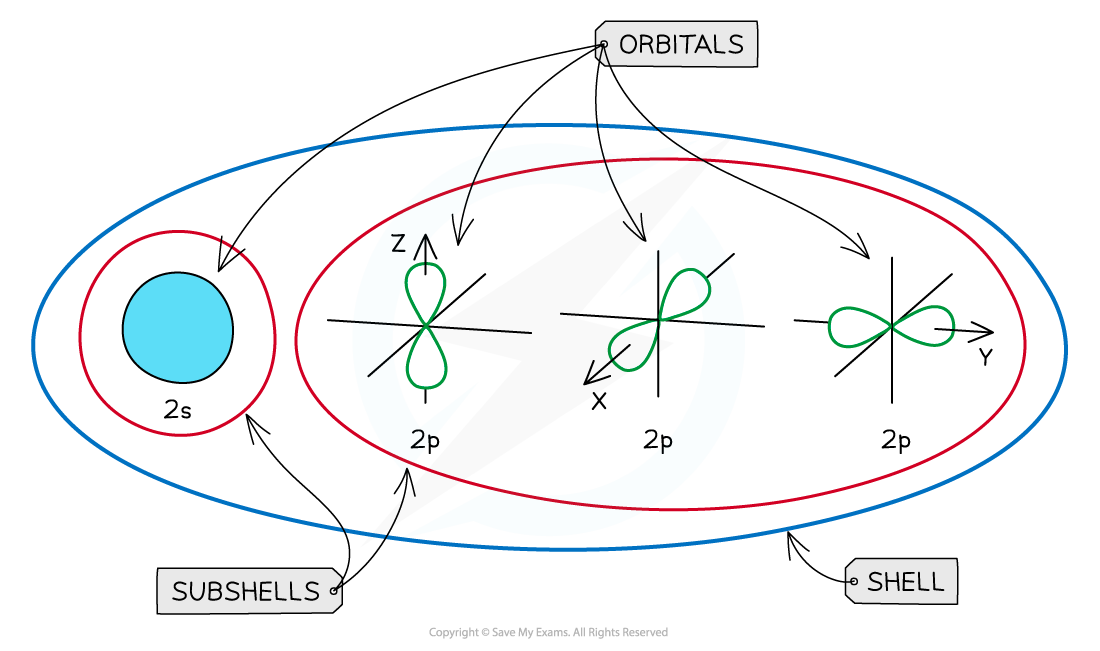An overview of the shells, subshells and orbitals in an atom

#### Ground state

• The ground state is the most stable electronic configuration of an atom which has the lowest amount of energy
• This is achieved by filling the subshells of energy with the lowest energy first (1s)
• The order of the subshells in terms of increasing energy does not follow a regular pattern at n = 3 and higher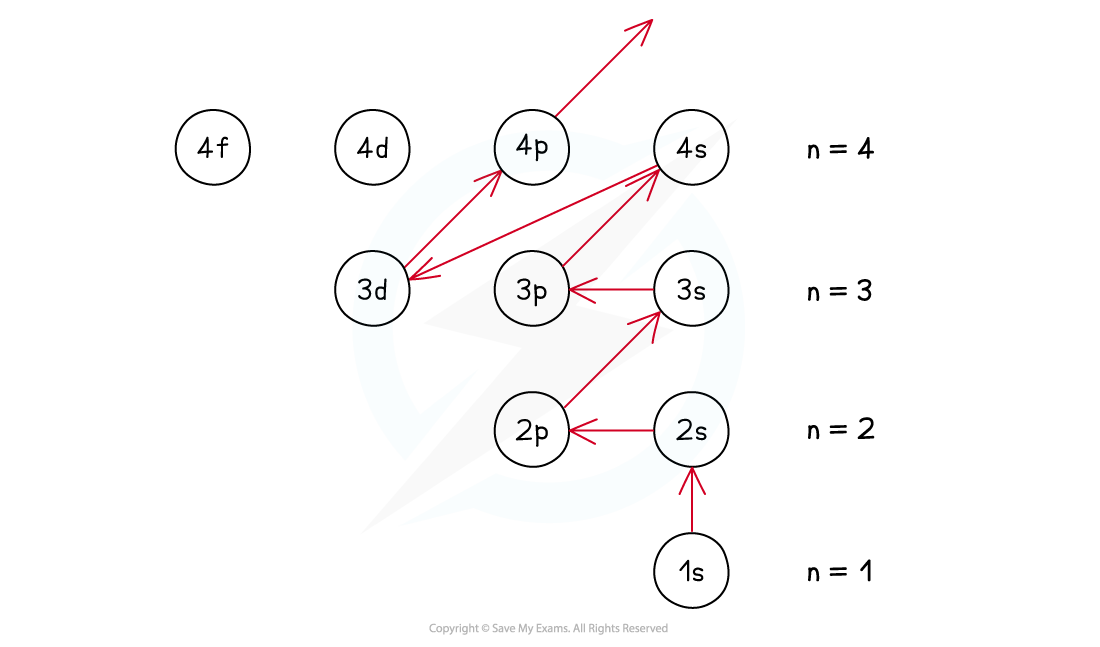The ground state of an atom is achieved by filling the lowest energy subshells first

### Electron Arrangement Summary

• Each shell can be divided further into subshells, labelled s, p, d and f
• Each subshell can hold a specific number of orbitals:
• s subshell : 1 orbital
• p subshell : 3 orbitals
• d subshell : 5 orbitals
• f subshell : 7 orbitals

• Each orbital can hold a maximum number of 2 electrons so the maximum number of electrons in each subshell are as follows:
• s : 1 x 2 = total of 2 electrons
• p : 3 x 2 = total of 6 electrons
• d : 5 x 2 = total of 10 electrons
• f : 7 x 2 = total of 14 electrons

Summary of the Arrangement of Electrons in Atoms Table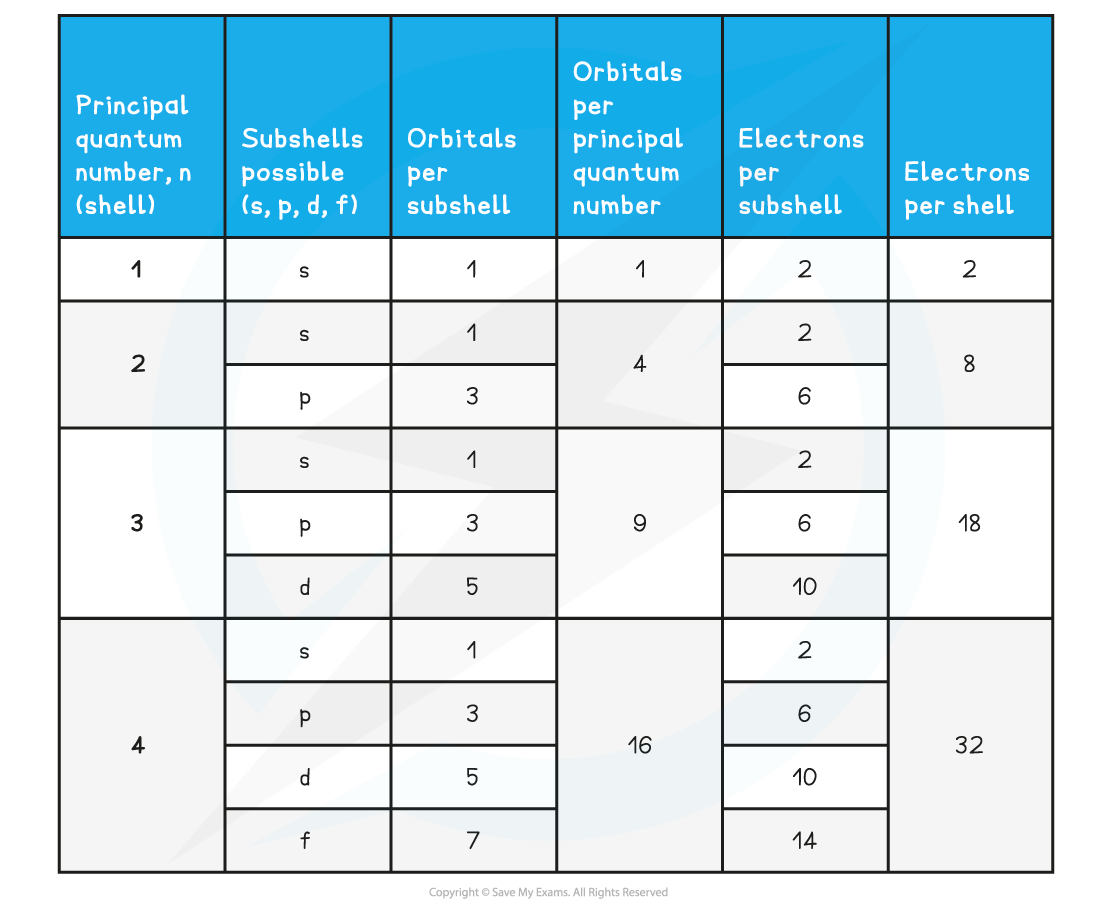#### Exam Tip

The three p orbitals are labelled px, py and pz, but you do not need to include this in your electron configurations!